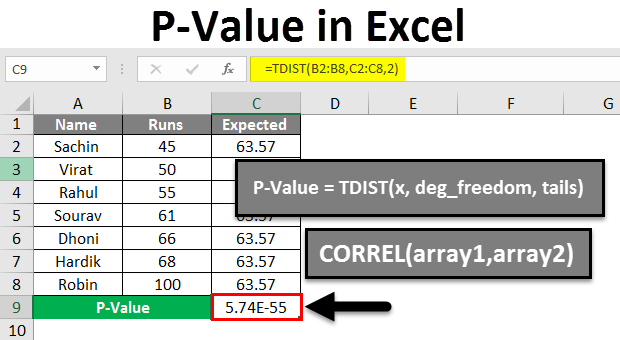Blog

# How to Calculate Statistical Significance in Excel?

Are you looking to understand how to calculate statistical significance in Excel? If so, you’ve come to the right place. With this article, you’ll learn the basics of how to use Excel to perform statistical tests, including how to calculate the significance of your findings. We’ll look at the different types of tests available and how to interpret the results. By the end of the article, you’ll have the tools you need to confidently analyze data and understand the results.## Understanding Statistical Significance in Excel

Statistical significance is a measure of the likelihood that the results of an experiment are real, rather than due to chance. In Excel, statistical significance is determined by comparing two sets of data. By understanding how to calculate statistical significance in Excel, researchers can determine if their experiments are producing meaningful results.

### What is Statistical Significance?

Statistical significance is a measure of the probability that the results of an experiment are real, rather than due to chance. It is used to determine if the results of an experiment are statistically significant, or if the results could have occurred by chance. Statistical significance is usually determined by comparing two sets of data. If the difference between the two sets of data is greater than what would be expected by chance, then the results are considered statistically significant.

### How to Calculate Statistical Significance in Excel

Calculating statistical significance in Excel requires first entering the two sets of data into the spreadsheet. Once the data has been entered, the user must then use the T-Test function to calculate the statistical significance. To use the T-Test function, the user must enter the two sets of data into separate columns and then use the T-Test formula to compare the two sets of data. The results of the T-Test will indicate if the difference between the two sets of data is statistically significant.

### Interpreting the Results

Once the T-Test has been completed, the user must interpret the results. The T-Test will produce two values, the T-Test statistic and the p-value. The T-Test statistic is a measure of the difference between the two sets of data. The p-value is a measure of the likelihood that the results are due to chance. If the p-value is less than 0.05, then the results are considered statistically significant.

### Using the Results

Once the user has determined if the results of the experiment are statistically significant, they can then use the results to draw conclusions about the experiment. If the results are statistically significant, then the user can conclude that the results are real and not due to chance. If the results are not statistically significant, then the user should consider other factors that may have influenced the experiment.

### Troubleshooting

If the user is having difficulty calculating statistical significance in Excel, there are several troubleshooting steps that can be taken. First, the user should make sure that the data is entered correctly into the spreadsheet. Second, the user should double-check the T-Test formula to make sure it is entered correctly. Finally, the user should check the p-value to make sure it is less than 0.05. If the p-value is greater than 0.05, then the results of the experiment are not statistically significant.

### Conclusion

Calculating statistical significance in Excel is a straightforward process. By understanding how to calculate statistical significance in Excel, researchers can determine if their experiments are producing meaningful results.

## Top 6 Frequently Asked Questions

### What is Statistical Significance?

Statistical significance is a measure of how likely a result is due to chance, rather than some underlying factor. It is used in scientific research to decide if an observed result is significant enough to reject the null hypothesis (the hypothesis that there is no real effect). Statistical significance can be determined by performing a statistical test, such as a t-test or chi-square test.

### What is the Formula for Calculating Statistical Significance?

The formula for calculating statistical significance is P-value = Probability of obtaining a result as extreme as the observed one, given that the null hypothesis is true. P-value is the probability of obtaining a result at least as extreme as the observed result, given that the null hypothesis is true.

### How to Calculate Statistical Significance in Excel?

In Excel, statistical significance can be calculated using the t-test or chi-square test. For the t-test, the formula is T = (Observed mean – Null mean) / Standard Error. For the chi-square test, the formula is Chi-square = (Observed – Expected)^2 / Expected.

### What Does a High Statistical Significance Mean?

A high statistical significance means that the observed result is very unlikely to have occurred by chance. This means that the null hypothesis is likely to be false, and that the observed result is likely due to some underlying factor.

### What Does a Low Statistical Significance Mean?

A low statistical significance means that the observed result is likely to have occurred by chance. This means that the null hypothesis is likely to be true, and that the observed result is likely not due to any underlying factor.

### What is the Significance Level?

The significance level is the probability of rejecting the null hypothesis when it is true. For example, a significance level of 0.05 means that there is a 5% chance of rejecting the null hypothesis if it is true. This means that if the observed result is significant at a significance level of 0.05, then it is likely that the null hypothesis is false.

In conclusion, calculating statistical significance in Excel can be a daunting task. However, with the right knowledge and understanding of the formulas and functions available, it can be a powerful tool to help you make data-driven decisions. By understanding the concept of statistical significance and the Excel functions associated with it, you can easily calculate and interpret data with confidence.